# A tungsten wire has a radius of 0.081 mm and is heated from 20.0 to 1315...

A tungsten wire has a radius of 0.081 mm and is heated from 20.0 to 1315 °C. The temperature coefficient of resistivity is α = 4.5 10-3 (C°)−1. When 110 V is applied across the ends of the hot wire, a current of 2.0 A is produced. How long is the wire? Neglect any effects due to thermal expansion of the wire.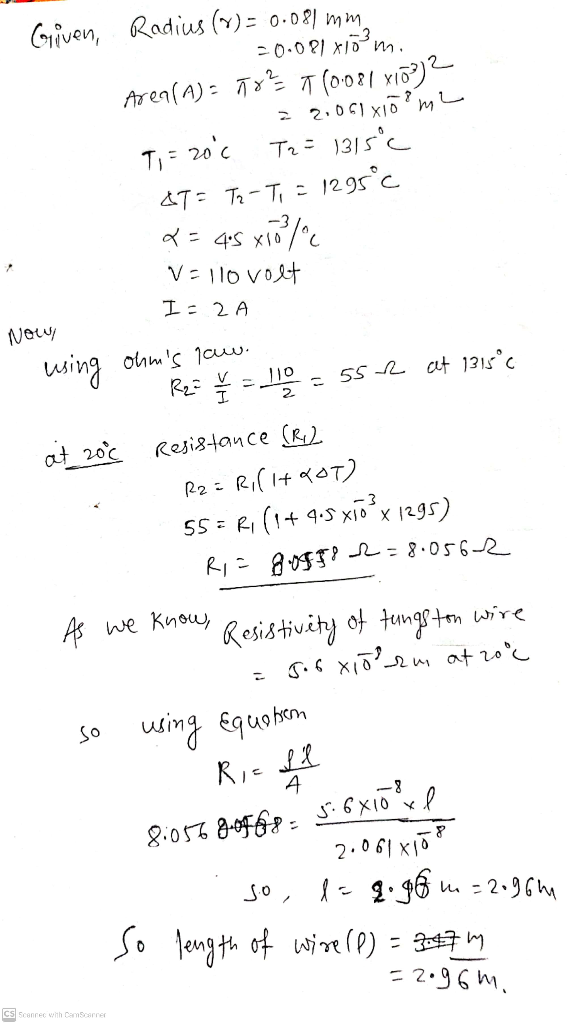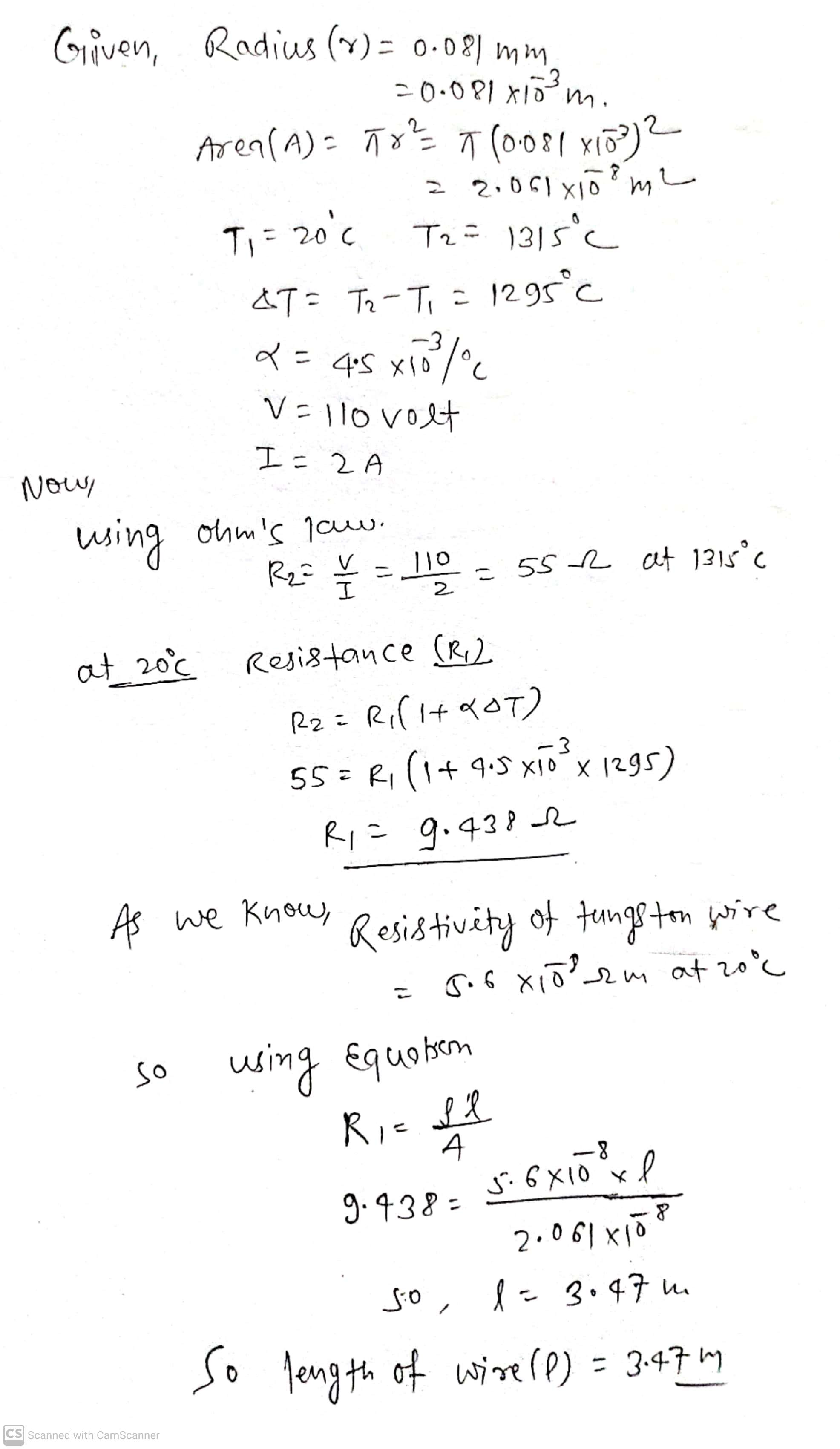hope you got. Please upvote my hardwork. Thanks

Given, Radius (%) = 0.081 mm -0.081 xidmi Area (A) = 782 T 10.081 +18)2 z 2,061 T, zo'c T2 = 1315c 8 XIO h, L &T= Tz-Ti = 1295°c x = 45 x 10 xio/c V = 110volt I = 2 Now using oum's low. v R2= 110 = 55 R at 1315°C I 2 -3 at 20°c Resistance (R.2 R2 = R(+4OT) 55= Ri (14 9.5 x16 x 1295) R1 = 9.4382 As we know, Resistivity of tungston wire gib xiorm at 20°C so using Equotson Ri= Il A s:6x10 l 9.438 8 2.061 x 10 l = 3.47 m so , So length of wire (1) = 3.47 m CS Scanned with CamScanner

##### Add Answer to: A tungsten wire has a radius of 0.081 mm and is heated from 20.0 to 1315...
Similar Homework Help Questions
• ### A tungsten wire has a radius of 0.061 mm and is heated from 20.0 to 1335...

A tungsten wire has a radius of 0.061 mm and is heated from 20.0 to 1335 °C. The temperature coefficient of resistivity is α = 4.5 10-3 (C°)−1. When 240 V is applied across the ends of the hot wire, a current of 1.2 A is produced. How long is the wire? Neglect any effects due to thermal expansion of the wire.

• ### A tungsten wire has a radius of 0.076 mm and is heated from 20.0 to 1305...

A tungsten wire has a radius of 0.076 mm and is heated from 20.0 to 1305 °C. The temperature coefficient of resistivity is α = 4.5 10-3 (C°)−1. When 120 V is applied across the ends of the hot wire, a current of 1.9 A is produced. How long is the wire? Neglect any effects due to thermal expansion of the wire.

• ### A tungsten wire has a radius of 0.087 mm and is heated from 20.0 to 1334...

A tungsten wire has a radius of 0.087 mm and is heated from 20.0 to 1334 oC. The temperature coefficient of resistivity is α = 4.5 x 10-3 (Co)-1. When 150 V is applied across the ends of the hot wire, a current of 1.3 A is produced. How long is the wire? Neglect any effects due to thermal expansion of the wire. help!!

• ### A tungsten wire has a radius of 0.089 mm and is heated from 20.0 to 1438...

A tungsten wire has a radius of 0.089 mm and is heated from 20.0 to 1438 oC. The temperature coefficient of resistivity is α = 4.5 x 10-3 (Co)-1. When 160 V is applied across the ends of the hot wire, a current of 1.4 A is produced. How long is the wire? Neglect any effects due to thermal expansion of the wire.

• ### A tungsten wire has a radius of 0.093 mm and is heated from 20.0 to 1333...

A tungsten wire has a radius of 0.093 mm and is heated from 20.0 to 1333 oC. The temperature coefficient of resistivity is α = 4.5 x 10-3 (Co)-1. When 130 V is applied across the ends of the hot wire, a current of 2.2 A is produced. How long is the wire? Neglect any effects due to thermal expansion of the wire.

• ### A tungsten wire has a radius of 0.078 mm and is heated from 20.0 to 1369...

A tungsten wire has a radius of 0.078 mm and is heated from 20.0 to 1369 oC. The temperature coefficient of resistivity is α = 4.5 x 10-3 (Co)-1. When 140 V is applied across the ends of the hot wire, a current of 2.2 A is produced. How long is the wire? Neglect any effects due to thermal expansion of the wire.

• ### A tungsten wire has a radius of 0.075 mm . The coefficient of resistivity is 5.6...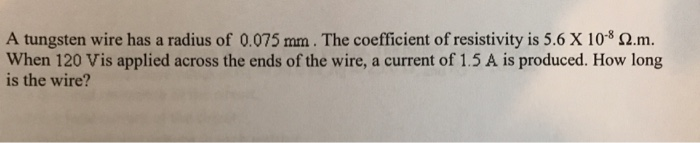A tungsten wire has a radius of 0.075 mm . The coefficient of resistivity is 5.6 X 10-8 Ω·m. When 120 Vis applied across the ends of the wire, a current of 1.5 A is produced. How long is the wire?

• ### A portion of Nichrome wire of radius 2.80 mm is to be used in winding a...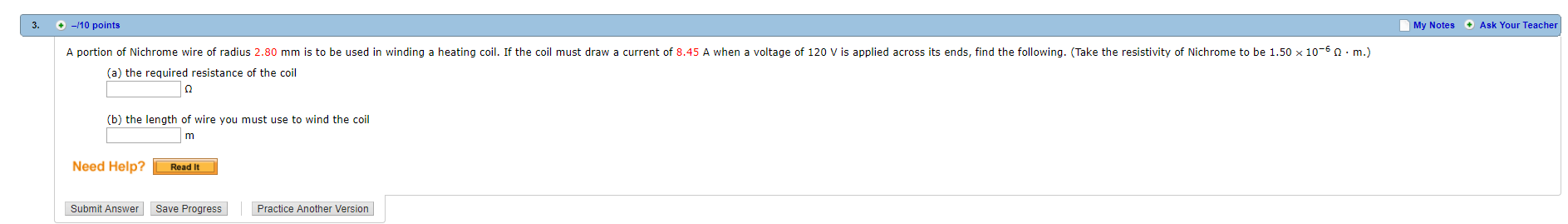A portion of Nichrome wire of radius 2.80 mm is to be used in winding a heating coil. If the coil must draw a current of 8.45 A when a voltage of 120 V is applied across its ends, find the following. (Take the resistivity of Nichrome to be 1.50 ? 10?6 ? · m.) 3. -10 points My Notes Ask Your Teacher A portion of Nichrome wire of radius 2.80 mm is to be used in winding a heating...

• ### The temperature coefficient of resistance α in equation R(T)=R0[1+α(T−T0)] equals the temperature coefficient of resistivity α...

The temperature coefficient of resistance α in equation R(T)=R0[1+α(T−T0)] equals the temperature coefficient of resistivity α in equation ρ(T)=ρ0[1+α(T−T0)] only if the coefficient of thermal expansion is small. A cylindrical column of mercury is in a vertical glass tube. At 20 ∘C, the length of the mercury column is 12.0 cm. The diameter of the mercury column is 1.6 mm and doesn't change with temperature because glass has a small coefficient of thermal expansion. The coefficient of volume expansion of...

• ### please do all parts a,b,c,d,e,f and g A wire is made of Tungsten with resistivity of...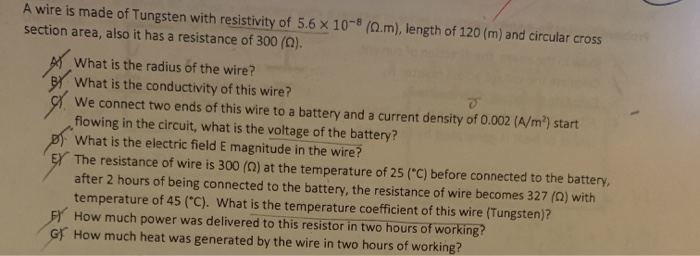please do all parts a,b,c,d,e,f and g A wire is made of Tungsten with resistivity of 5.6 x 10-8 (0.m), length of 120 (m) and circular cross section area, also it has a resistance of 300 (0). What is the radius of the wire? BY What is the conductivity of this wire? We connect two ends of this wire to a battery and a current density of 0.002 (A/m”) start flowing in the circuit, what is the voltage of the...

Free Homework App